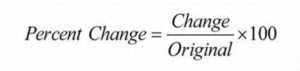# MS Math – Nov 15-19, 20216th Grade Math – Mrs. Evans

This week we will finish with graphing rational numbers in Chapter 3 with a test on Wednesday.  We will begin to investigate ratios, rates and unit rates as we work into Chapter 4.  Here is a video about ratios and rates:

Math Grade Math – Mrs. Evans and Mrs. VonFeldt

This week, we will finish our study of proportional relationships with a heavy quiz on  Thursday.  We have learned a lot in this module and will be able to apply it throughout the rest of the year.  In Module 5 we will plunge into studying about percents and how to determine a percent change.  The percent change is defined as follows:We will be determining the markup and markdown on quantities using percents.  Here is a video about percent change to introduce you to this useful concept.

8th Grade Math – Mrs. Ernest

Algebra – Mrs. VonFeldt

There will be a Chapter 3 test on Thursday, November 18. It will cover graphing linear equations. In Chapter 4 we will look at ways to find the equation of a line when you have different pieces of information. How can you find an equation when you have two points? A point and a slope? A y-intercept and a point? Students are getting very familiar with the different representations of linear situations. They can now graph directly from an equation, create a table from an equation or graph, and find an equation directly from a graph.

© 2022 Assumption Catholic School | 2116 Cornwall Ave, Bellingham, WA 98225
Phone: 360.733.6133 | Fax: 360.647.4372
Email: theoffice@school.assumption.org
Dashboard | Web design and development by Olywebdev.com